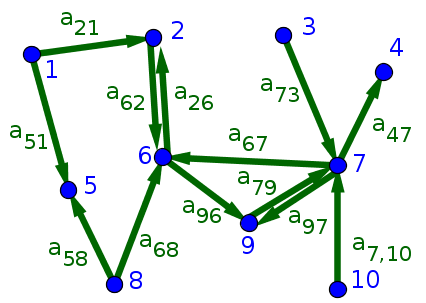# Math Insight

The adjacency matrix for a network of $N$ nodes is a matrix of ones and zeros where a one indicates the presence of the corresponding edge in the network. Unfortunately, if the network is directed, there exist opposite conventions for how to define the adjacency matrix. We use the convention of reading indices from right to left so that we define $A$ by \begin{gather*} a_{ij} \nobreak{=} \begin{cases} 1 & \text{if there is an edge from node $j$ to node $i$}\\ 0 & \text{otherwise.} \end{cases} \end{gather*}